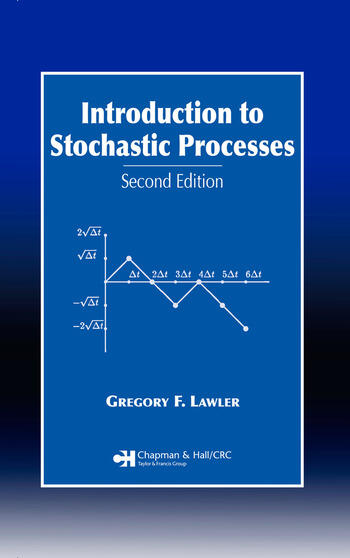# Introduction to Stochastic Processes

## 2nd Edition

Gregory F. Lawler

Chapman and Hall/CRC
Published May 16, 2006
Textbook - 248 Pages - 13 B/W Illustrations
ISBN 9781584886518 - CAT# C651X
Series: Chapman & Hall/CRC Probability Series

For Instructors Request Inspection Copy

USD\$110.00

FREE Standard Shipping!

## Preview

### Summary

Emphasizing fundamental mathematical ideas rather than proofs, Introduction to Stochastic Processes, Second Edition provides quick access to important foundations of probability theory applicable to problems in many fields. Assuming that you have a reasonable level of computer literacy, the ability to write simple programs, and the access to software for linear algebra computations, the author approaches the problems and theorems with a focus on stochastic processes evolving with time, rather than a particular emphasis on measure theory.

For those lacking in exposure to linear differential and difference equations, the author begins with a brief introduction to these concepts. He proceeds to discuss Markov chains, optimal stopping, martingales, and Brownian motion. The book concludes with a chapter on stochastic integration. The author supplies many basic, general examples and provides exercises at the end of each chapter.

New to the Second Edition:
• Expanded chapter on stochastic integration that introduces modern mathematical finance
• Introduction of Girsanov transformation and the Feynman-Kac formula
• Expanded discussion of Itô's formula and the Black-Scholes formula for pricing options
• New topics such as Doob's maximal inequality and a discussion on self similarity in the chapter on Brownian motion

Applicable to the fields of mathematics, statistics, and engineering as well as computer science, economics, business, biological science, psychology, and engineering, this concise introduction is an excellent resource both for students and professionals.
• #### Instructors

We provide complimentary e-inspection copies of primary textbooks to instructors considering our books for course adoption.